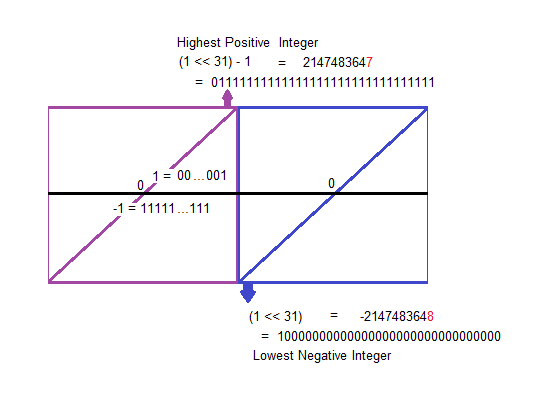Data Structuresand Algorithms Good OldJava AdvancedInterview Topics Cloud andDatabases Web Designand Development Must-knowTools Good ToKnow

## Bitwise representation of int and long boundaries

Boundaries:
 ``` Integers: 3 = 00000000000000000000000000000011 2 = 00000000000000000000000000000010 1 = 00000000000000000000000000000001 0 = 00000000000000000000000000000000 -1 = 11111111111111111111111111111111 -2 = 11111111111111111111111111111110 -3 = 11111111111111111111111111111101 2147483647 = 01111111111111111111111111111111 -2147483648 = 10000000000000000000000000000000 Longs: 3 = 0000000000000000000000000000000000000000000000000000000000000011 2 = 0000000000000000000000000000000000000000000000000000000000000010 1 = 0000000000000000000000000000000000000000000000000000000000000001 0 = 0000000000000000000000000000000000000000000000000000000000000000 -1 = 1111111111111111111111111111111111111111111111111111111111111111 -2 = 1111111111111111111111111111111111111111111111111111111111111110 -3 = 1111111111111111111111111111111111111111111111111111111111111101 9223372036854775807 = 0111111111111111111111111111111111111111111111111111111111111111 -9223372036854775808 = 1000000000000000000000000000000000000000000000000000000000000000 ```Code:
```
public class BitsOfNegativeNumber
{

public static void main(String[] args)
{
for (int num = 3; num >= -3; num--)
printBinary(num);

System.out.println();

int n = (1<<31) - 1;
printBinary (n);
n++;
printBinary(n);

System.out.println("\n\nLongs\n");
for (long num = 3; num >= -3; num--)
printLongToBinary(num);

System.out.println();

long n2 = (1L << 63) - 1;
printLongToBinary (n2);
n2++;
printLongToBinary(n2);
}

static void printBinary(int num)
{
int n = num;
String s = "";
for (int i=0; i<32;i++)
{
s = (n&1) + s;
n = n >> 1;
}
System.out.println(num + " = " + s);
}

static void printLongToBinary(long num)
{
long n = num;
String s = "";
for (int i=0; i<64;i++)
{
s = (n&1) + s;
n = n >> 1;
}
System.out.println(num + " = " + s);
}
}

```

Like us on Facebook to remain in touch
with the latest in technology and tutorials!

Got a thought to share or found a
bug in the code?
We'd love to hear from you:

 Name: Email: (Your email is not shared with anybody) Comment: